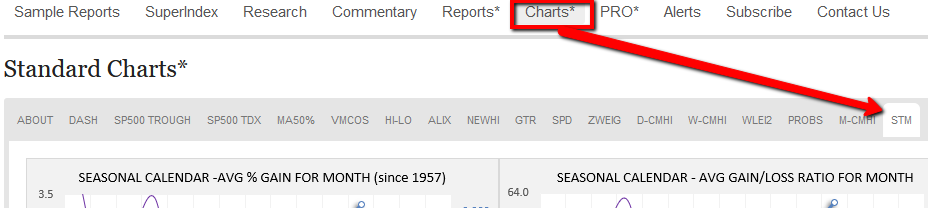# Analyzing SP-500 Seasonal Trends

For this research, we examine annual, bi-annual, tri-annual and quadrennial cyclical seasonal characteristics of SP500 monthly closing prices since 1957 and show how to combine all these cycles into a single powerful seasonal indicator.

The annual cycle consists of a single phase of 12 months which comprise the historical statistical record of 63 cycles. The bi-annual cycle consists of two phases of 12 months each which comprise the historical statistical record of 32 cycles. The tri-annual cycle consists of three phases of 12 months each which comprise the historical statistical record of 21 cycles. The quadrennial (otherwise known as the presidential election cycle) consists of 4 phases of 12 months each which comprise the historical statistical record of 15 cycles since 1957.

There are definite, persistent cyclical seasonal patterns which are identified when examining three characteristics of statistical monthly performances of the SP500 during the cycle phases, namely

1. Average % gain for the month
2. % of positive months
3. total gains in positive months divided by total losses in negative months (gain/loss ratio)

The three items above (statistical performance characteristics or SPC’s for short) provide important clues as to the expected magnitude, success rate and risk/reward of  projected outcomes of upcoming months forecast by a particular seasonal pattern phase.

For each month in each phase of each of the 4 cyclical cycles we compute a composite score from the 3 SPC’s based on an averaged ranking scoring methodology to attempt to provide an overall assessment of the statistical significance of the month, taking all cycles into account. Months with high composite scores can be attached high confidence of positive outcomes whilst months with low composite scores can be attached lower confidence.

### Annual Cycle

This is a cycle with 1 phase of 12-months duration, comprising 63 sample sets since 1957. As of this writing we are in month 12 (December). We see that the 63 Decembers since 1957 posted an average gain of 1.29%, with 73% being positive and gains exceeding losses by a factor of 2.85 times to 1.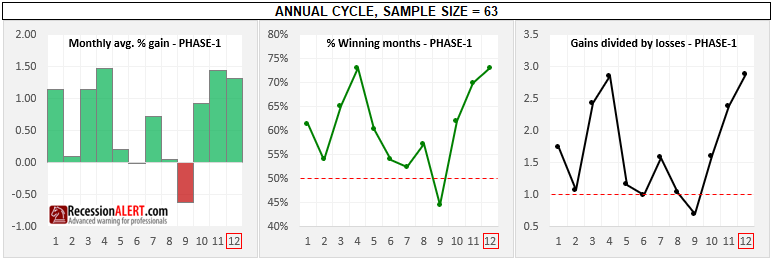To compile a single composite score for each month we must compile statistical ranking scores for each of the 3 SPC’s in each month and average them up. For each SPC the annual cycle provides 1-phase of 12 monthly readings, the bi-annual cycle provides 2-phases of 12 monthly readings totaling 24 monthly readings, the tri-annual cycle provides 3-phases of 12 monthly readings for a total of 36 monthly readings and the quadrennial cycle provides 4-phases of 12 monthly readings for a total of 48 monthly readings. For the ranking exercise we thus have a total of 12+24+36+48 = 120 total readings.

As we have shown above, the Annual Cycles’ December month has an average monthly gain of 1.29%. This happens to be ranked 30 best monthly gain from the 120 total readings across all cycles. We can compute this to a more intuitive percentage score with the formula (121-rank)/120 = (121-30)/120 = 0.758. This means that the December month average gains in the annual cycle is better than 75.8% of all average monthly gains witnessed across the 1,2,3 and 4 year cyclical seasonal patterns.

The Annual Cycles’ December month has a 73% positive gain rating which happens to be ranked 19th best win-rate across the 120 total readings across all cycles. We compute the score (121-19)/120=0.85 which means the annual cycles December month has a win-rate that is better than 85% of the sample.

The Annual Cycles’ December months has a gain/loss ratio of 2.85 which happens to be ranked 25th best gain/loss ratio across the 120 total readings across all cycles. Its gain/loss score is thus (121-25)/120 = 0.8 which implies it is better than 80% of the entire sample set across all seasonal cycles.

To arrive at a composite score for the Annual Cycles’ December month we take the 3 SPC scores and average them, namely (0.758+0.85+0.8)/3=0.802. We can thus deduce that the Annual Cycles’ December months is a very strong statistical reading with a high implied gain, win rate and risk/reward ratio. We may be inclined to go long the SP500 with a 2X leveraged position in December months if we are following the annual statistical seasonal cycle.

Following the above methodology for the January through December months SPC’s from the Annual Seasonal Cycle we get the below scoring profile: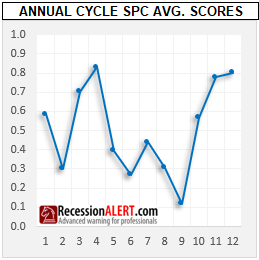We deduce that suggestions about positive predicted SP500 returns from the Annual Cycle for March, April , Nov and Dec could be taken seriously as they are statistically significant. The bearish Sept months could also be taken seriously with a short position on the SP500.

### Bi-annual cycle

This is a cycle with 2 phases of 12-months duration, comprising 32 sample sets since 1957. As of this writing, it is in month 12 of Phase-1.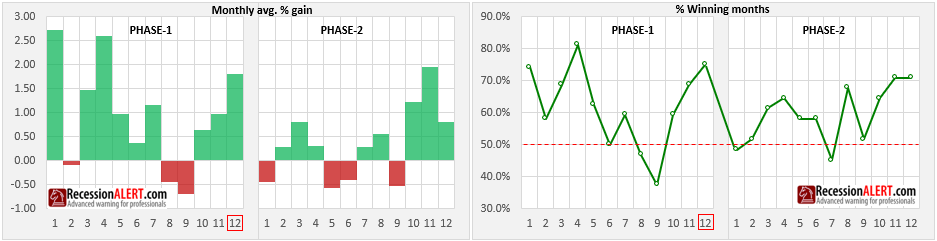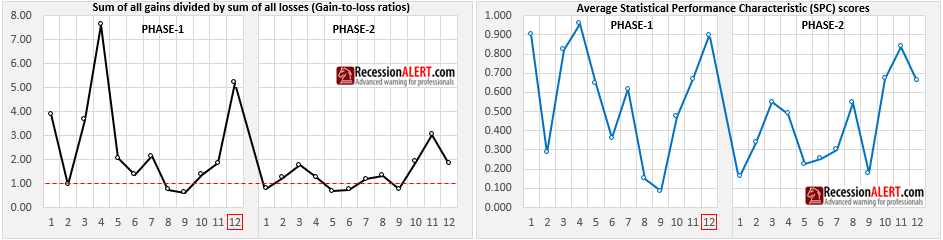It is clear that Phase-1 of the bi-annual cycle is statistically a better performer than the second phase with January, April and December of Phase-1 being among the best performing months of the entire sample set across all seasonal cycles (scores > 0.9)

### Tri-annual cycle

This is a cycle with 3 phases of 12-months duration, comprising 21 sample sets since 1957. The statistical performance factors (SPF’s) appear below: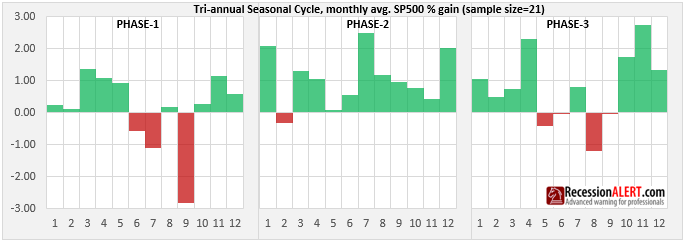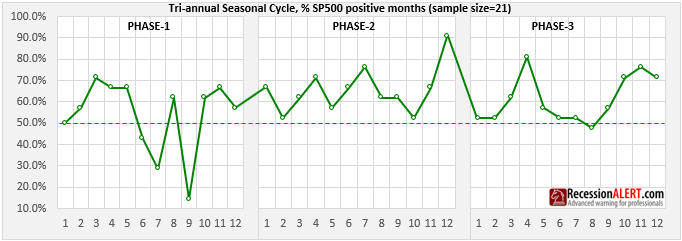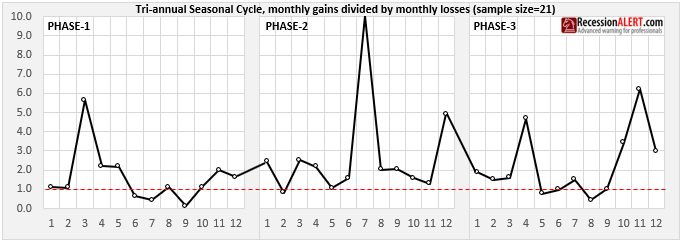We see a lot of individual monthly powerful statistical significances dispersed among the different SPF’s, so combining them together in our averaged ranking scoring methodology provides an easier way to see all the statistically significant months: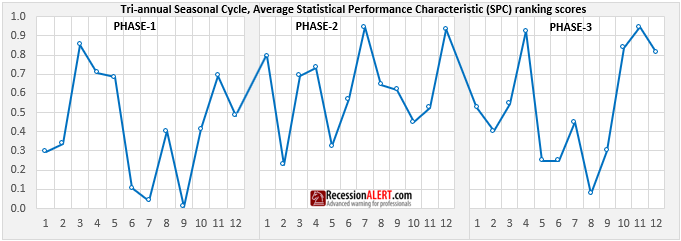This is a cycle with 4 phases of 12-months duration, comprising 15 sample sets since 1957. This is a particularly famous and well researched stock market cycle, otherwise known as the U.S Presidential Election Cycle: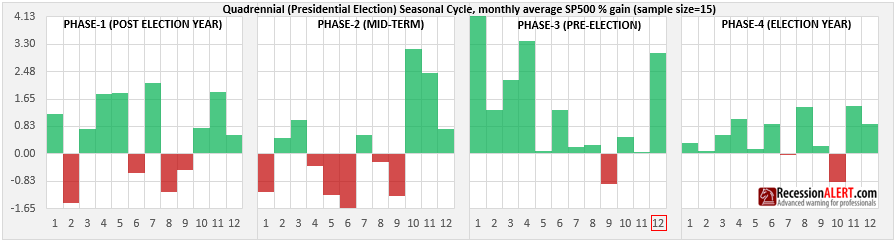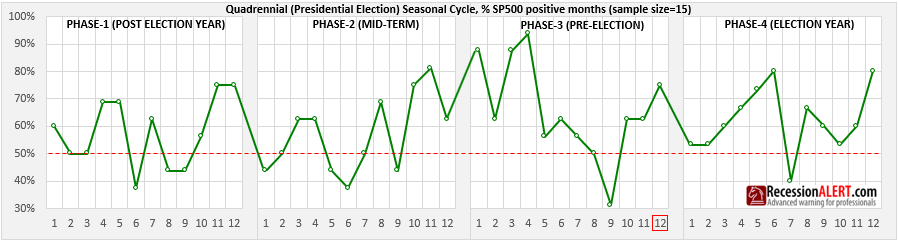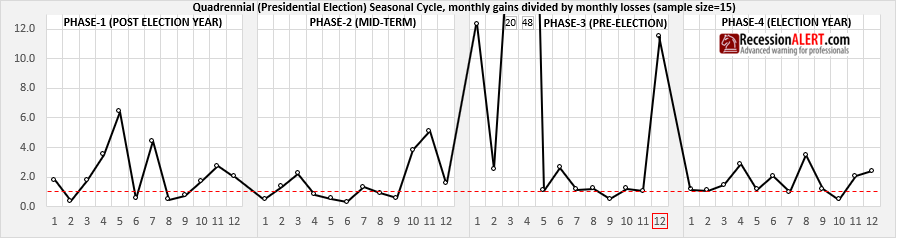Again, we see a lot of individual monthly powerful statistical significances dispersed among the different SPF’s, so combining them together in our averaged ranking scoring methodology provides an easier way to see all the statistically significant months taking all factors into account: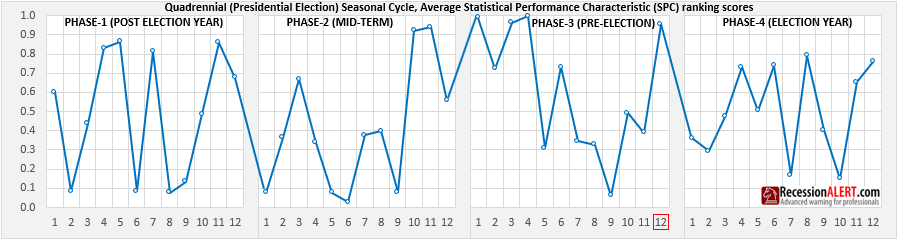We notice a lot of very high confidence seasonality outcomes (score = 0.8 and above, 0.2 and below), much more than the annual, bi-annual and tri-annual cycles.

### Combined Cycle

We can take the average of the 4 different seasonal cycles’ SPC ranking scores to achieve a combined seasonal cycle that considers multiple cyclical seasonal patterns in the SP500. The idea is similar to that of multiple waves of different frequency, combining now and again to provide amplified wave height and hopeful provide us with better information on statistical significance and likelihood of future outcomes.

When combining cycles to calculate averages or ranking scores, we use the method depicted below using the 1/2/3 year seasonal cycles as example, with time marching from left to right. The first digit represents the month of the year and the second digit the phase the cycle is currently in: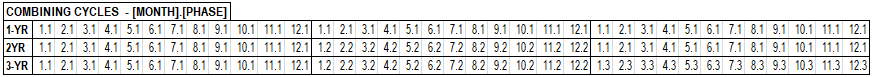The chart below left shows how this is accomplished and buy+hold out-performance can be achieved across all long, short, leveraged or non-leveraged applications of the combined composite ranking score/signal as shown on the 10-year performance chart on the bottom right: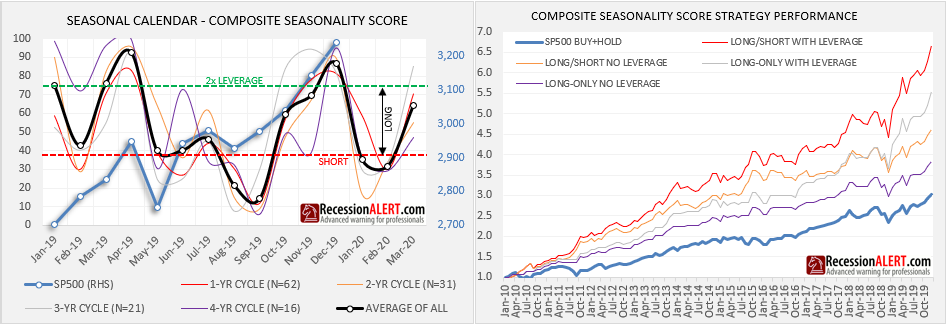You will note that the top-left chart also provides expected statistical outcomes in the months ahead, which is very useful for planning purposes.

The seasonal strategy goes as follows:

1. Go short when the signal is < 37 (red dotted line above)
2. Go long when the signal is >=37 and <=74
3. Apply 2x leverage to positions when signal is >74

You could of course elect to ignore shorts (going to cash instead) or not using leverage. All the possible combinations of the use of the seasonal strategy with or without shorting or leverage are shown in the top right chart above.

One does not have to deploy a market timing strategy to use the seasonality score however. One could simply use the composite ranking signal to determine statistical significance of upcoming months to apply some risk management to ongoing operations or strategies. Naturally when the signal is above 80% or below 20% you then know you have rare events of high statistical significance.

The seasonal charts we provide subscribers also enable you to inspect specific statistical performance characteristics that may be of interest, together with their averages across multiple cycles, such as shown on the two charts below: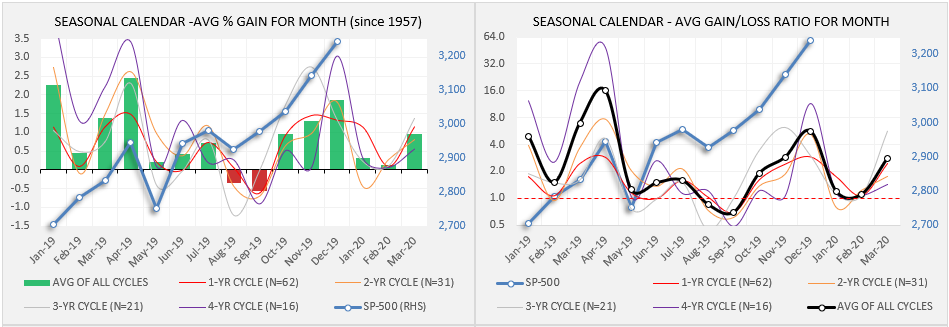The seasonal charts are available to subscribers in the CHARTS>STM section: